# Physics - Electromagnetism - RC circuit exercises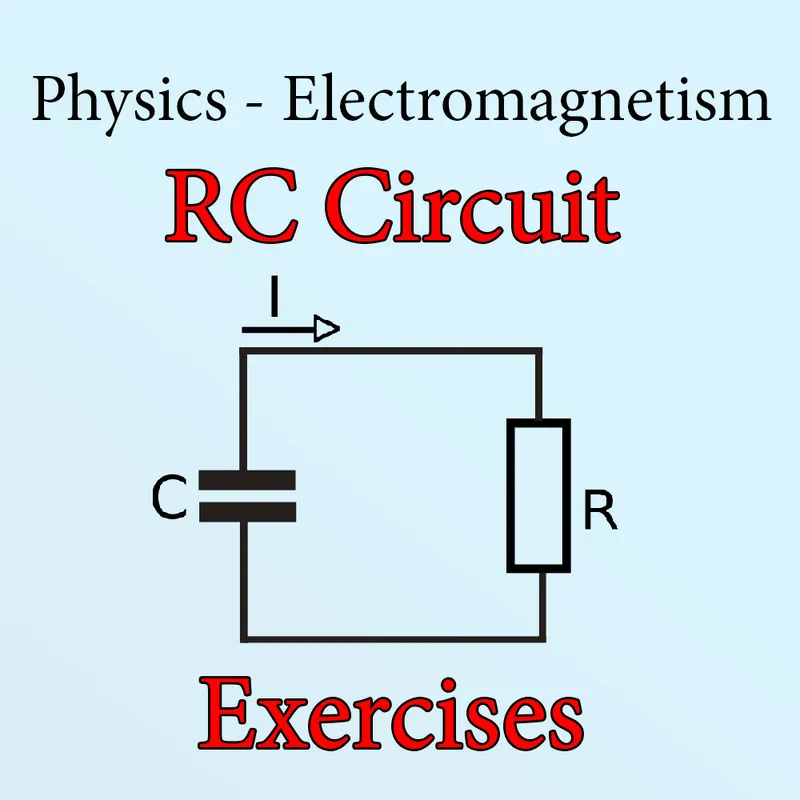Custom thumbnail using:

## Introduction

Hello it's a me again Drifter Programming! Today we continue with Electromagnetism to get into RC circuit exercises. You should read the previous article of mine if you haven't already, cause this post will be based on the R-C circuit theory we covered there. So, without further do, let's get straight into it!

## Kirchhoff's law example:

Let's tweak Example#1 of https://web.pa.msu.edu...

Suppose that we have the following circuit: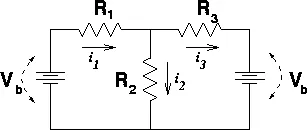Image source: https://web.pa.msu.edu/

Let's say that all resistors have the same resistance R = 15Ω and Vb = 10V. What is the current that flows through all the resistors of the circuit?

Solution:

As independent variable we will consider the current. In the example of the website they apply the KVL law for the left and right loops. So, let's apply it for the "big" loop outside and for the left loop. For both we will consider clockside movement. That way we end up with: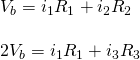Applying the KCL law the current i3 is equal to:By substituting the current i3 in the second equation and adding them together we end up with the current i1: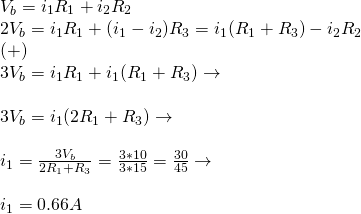That way the currents i2 and i3 are:That my friends is why you never change the numbers of examples :P The funny thing tho is that the currents are not negative, which means that the direction shown in the diagram is correct for our application!

## Simple charging example:

Suppose a simple RC circuit with a battery, resistor, capacitor and switch like the following:Image source:This example will be based on the example at: https://www.electronics-tutorials.ws/rc/rc_1.html.

Suppose that the voltage is V = 4V (+ pole on top, - on bottom), that the resistance of the resistor is R = 70kΩ and that the capacitance of the capacitor is C = 1500μF (tweaked the numbers a little bit). The switch is being closed at t = 0.

Let's calculate:

1. The time constant τ
2. The voltage across the capacitor (Vc) after 0.7τ seconds
3. The voltage across the capacitor (Vc) after 1τ seconds
4. The time taken for a "full charge"
5. The voltage across the capacitor after 100 seconds

Solution:

1.

The time constant is given by τ = RC and so is equal to: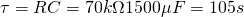2.

From the tables we know that the voltage is at ~50% of maximun after 0,7τ and so: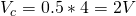3.

From the tables we know that after 1τ the value is ~63% of maximum and so: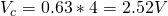4.

To fully charge a capacitor we know that it takes 5τ seconds approximately (steady state period) and so the time taken is: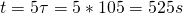5.

For 100 seconds we don't have an easy and direct way and so we have to use the equation v(t). This means that the voltage after 100 seconds is:Because τ = 105seconds we see that our calculated value is very close to the value we calculated for 3. And so whenever there is some "known" result we use the percentage from the table, else we have to use the formula/equation which works for any time t.

## Simple discharging example:

Suppose the moment of the previous example, where the capacitor is fully charged. The total charge reached is of course equal to: Q = CV = 1500μF*4 = 6mC. Let's say that we "magically" get rid of the battery and so only the resistor and capacitor remain and also the switch, which at first (t = 0) is open.

I will be based on the example at: https://www.electronics-tutorials.ws/rc/rc_2.html cause it contains good questions. Of course the time constant is the same as before (τ = 105s).

Let's calculate:

1. The voltage across the capacitor after 0.7τ seconds
2. The voltage across the capacitor after τ seconds
3. How long it takes for the capacitor to "fully discharge"
4. The charge after 300 seconds (approximately 3τ)

Solution:

1.

From the table we know that after 0.7τ seconds the voltage has dropped by ~50% and so: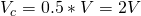2.

From the table we know that after τ seconds we have discharged to 36,6% of maximum and so: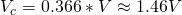3.

The time taken for a full discharge is approximately equal to 5τ which is:At this point the voltage and current are considered zero!

4.

For 300seconds, which is not a direct multiple of the time constant (τ) we don't have a direct percentage and so we have to use the equation v(t) which gives us: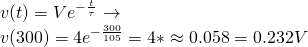t = 300 seconds are very close to 3τ, because τ = 105s ~ 100 and so the value we calculated is a little bit more then 5% which is 0.2V given by the table for 3τ.

## Battery with internal resistance:

Let's consider the circuit of before, but now the battery will also have an internal resistance of r = 1kΩ. I got the idea for internal resistance from the example at: https://phys.libretexts.org/, which is an application for relaxation oscillators!

So, let's calculate:

1. The new time constant τ
2. The "full charge" and "full discharge" time
3. The voltage after τ seconds of charging from complete discharge
4. The voltage after τ seconds of discharging from a complete charge

Solution:

1.

The total resistance of the circuit will now of course be Rtot = r + R = 1 + 70 = 71kΩ. That way the time constant now changes and is equal to: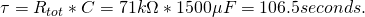2.

The full charge and full discharge time is approximately 5τ (steady state period) and so equal to: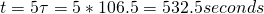3.

From the table we know that after τ seconds we will reach 63.2% of the maximum voltage (supplied by the battery) and so: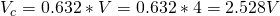4.

From the table we know that after τ seconds we will reach a voltage 36.6% that of the initial voltage we had before discharging and so: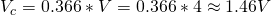We can see that the voltage doesn't change at all. Of course after the same amount of time the first circuit would be "fully charged" (and charged in general) much quicker, but when talking about τ seconds the voltage is exactly the same...

More examples can be found in the following:

More great problems on which you can practice on can be found here:

It gives you the solutions/answers, which means that you can compare your results!

## REFERENCES:

Some Mathematical equations that I had in this post where drawn using quicklatex

### Electric fields:

Getting into Electromagnetism -> electromagnetim, electric charge, conductors, insulators, quantization

Coulomb's law with examples -> Coulomb's law, superposition principle, Coulomb constant, how to solve problems, examples

Electric fields and field lines -> Electric fields, Solving problems around Electric fields and field lines

Electric dipoles -> Electric dipole, torque, potential and field

Electric charge and field Exercises -> examples in electric charges and fields

### Electric flux:

Electric flux and Gauss's law -> Electric flux, Gauss's law

Applications of Gauss's law (part 1) -> applying Gauss's law, Gauss applications

Applications of Gauss's law (part 2) -> more Gauss applications

Electric flux exercises -> examples in electric flux and Gauss's law

### Electric potential:

Electric potential energy -> explanation of work-energy, electric potential energy

Calculating electric potentials -> more stuff about potential energy, potential, calculating potentials

Millikan's Oil Drop Experiment -> Millikan's experiment, electronvolt

Cathode ray tubes explained using electric potential -> cathode ray tube explanation

Electric potential exercises (part 1) -> applications of potential

Electric potential exercises (part 2) -> applications of potential gradient, advanced examples

### Capacitance:

Capacitors (Condensers) and Capacitance -> Capacitors, capacitance, calculating capacitance

How to solve problems around Capacitors -> combination, solving problems, simple example

Electric field energy and density -> Electric field energy, energy density

Dielectric materials -> Dielectrics, dielectric constant, permittivity and strength, how to solve problems

Electric capacitance exercises -> examples in capacitance, energy density and dielectrics

### Current, resistance and EMF:

Electric current -> Electric current, current density

Electrical resistivity and conductivity -> Electrical resistivity, conductivity, thermal coefficient of resistivity, hyperconductivity

Electric resistance -> Resistance, temperature, resistors

Electromotive Force (EMF) and Internal resistance -> Electromotive force, internal resistance

Power and Wattage of Electronic Circuits -> Power in general, power/wattage of electronic circuits

Electric current, resistance and emf exercises -> exampes in all those topics

### Direct current (DC) circuits:

Resistor Combinations -> Resistor combinations, how to solve problems

Kirchhoff's laws with applications -> Kirchhoff's laws, how to solve problems, applications

Electrical measuring instruments -> what are they?, types list, getting into some of them, an application

Electronic circuits with resistors and capacitors (R-C) -> R-C Circuit, charging, time constant, discharging, how to apply

And this is actually it for today's post and I hope that you learned something!

Next time we will start getting into Magnetism, starting off with Magnetic fields!

C ya!

H2
H3
H4
3 columns
2 columns
1 column
1 Comment